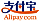Borítókép a The Intricate Nature of Mathematics - hoz
Könyv címe:

# The Intricate Nature of Mathematics

LAP LAMBERT Academic Publishing (11.07.2013 )Érvényes utalvány
ISBN-13:

### 978-3-659-42443-4

ISBN-10:
3659424439
EAN:
9783659424434
Könyv nyelve:
angol
Ismertető:
This book emerged from a set of lecture notes used by one of the authors to teach a 200 level course in Nature of Mathematics. The course was introduced as a bridge from traditional Calculus I, II, III courses to higher level courses of Mathematics, such as Abstract Algebra and Advanced Calculus. The book introduces basic notions from set theory, symbolic logic, functions and relations, number theory, combinatorics and graph theory. It also gives an introduction to more abstract mathematical proofs. The techniques discussed in the book include: direct proof, in particular proof by enumeration of cases, proof by contradiction, proof by mathematical induction, proofs using Well Ordering Principle, pigeonhole principle, inclusion-exclusion principle and coloring arguments.Besides a transitional course, the book can be used to teach a course in discrete mathematics, combinatorics, or even a general education course in mathematics to a class of motivated students.
Weboldal:
https://www.lap-publishing.com/
Szerezte:
Viorel Nitica, Catalin Nitica
Oldalszám:
204
11.07.2013
Raktárkészlet:
Raktáron
Kategória:
Matematika
Ár:
69,90 €
Kulcsszavak:
general education, Discrete Mathematics, nature of mathematics### Kategóriák